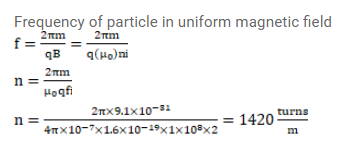# A tightly-wound, long solenoid carries a current of

Question:

A tightly-wound, long solenoid carries a current of $2.00 \mathrm{~A}$. An electron is found to execute a uniform circular motion inside the solenoid with a frequency of $1.00 \times 10^{8} \mathrm{rev} / \mathrm{s}$. Find the number of turns per meter in the solenoid.

Solution: